## RS Aggarwal Class 7 Solutions Chapter 2 Fractions Ex 2D

These Solutions are part of RS Aggarwal Solutions Class 7. Here we have given RS Aggarwal Solutions Class 7 Chapter 2 Fractions Ex 2D.

Other Exercises

OBJECTIVE QUESTIONS
Mark (√) against the correct answer in each of the following:
Question 1.
Solution:
(c)
Denominator in (a) and (b) is 10
These are decimal fractions
But denominator of (c) is 3
$$\frac { 10 }{ 3 }$$ is a vulgar fraction

Question 2.
Solution:
(c)
$$\frac { 7 }{ 10 }$$ and $$\frac { 7 }{ 9 }$$ are proper fractions as each of these have numerator less than its denominator
$$\frac { 9 }{ 7 }$$ is improper fraction

Question 3.
Solution:
(a)
$$\frac { 105 }{ 112 }$$ is reducible fraction because HCF 112 of 105 and 112 is 7

Question 4.
Solution:
(c)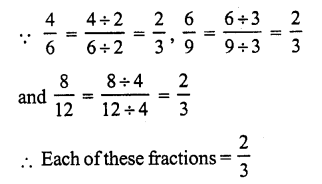Question 5.
Solution:
(c)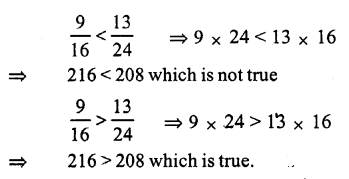Question 6.
Solution:
(d)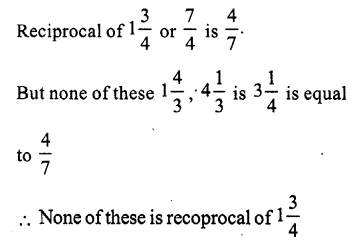Question 7.
Solution:
(c)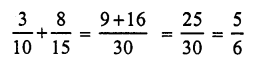Question 8.
Solution:
(d)Question 9.
Solution:
(d)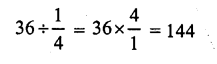Question 10.
Solution:
(b)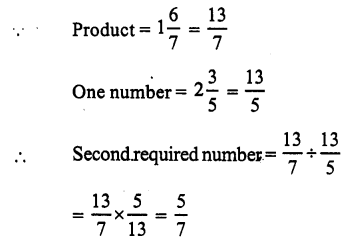Question 11.
Solution:
(d)Question 12.
Solution:
(c)Question 13.
Solution:
(d)Question 14.
Solution:
(d)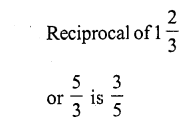Question 15.
Solution:
(b)
The correct statement will beQuestion 16.
Solution:
(c)
A car runs in 1 litre of petrol = 16 km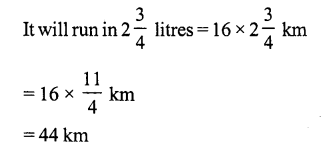Question 17.
Solution:
(a)Hope given RS Aggarwal Solutions Class 7 Chapter 2 Fractions Ex 2D are helpful to complete your math homework.

If you have any doubts, please comment below. Learn Insta try to provide online math tutoring for you.